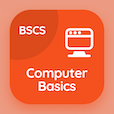Business Mathematics Quiz PDF - Complete

# Characteristics of Exponential Functions Multiple Choice Questions p. 32

Study Characteristics of Exponential Functions multiple choice questions and answers, characteristics of exponential functions quiz answers PDF 32 to study Business Mathematics course online. Exponential and Logarithmic Functions MCQ trivia questions, characteristics of exponential functions Multiple Choice Questions (MCQ) for online college degrees. "Characteristics of Exponential Functions MCQ" PDF eBook: single payment computations, second degree equation in one variable, types of functions, linear functions in maths, characteristics of exponential functions test prep for BA in business administration.

"The function which describes increase of 3% in child death rate is classified as" MCQ PDF: life function, exponential function of decline, exponential function of growth, and exponential decay function for online business administration degree. Learn exponential and logarithmic functions questions and answers to improve problem solving skills for online business management degree programs.

## Characteristics of Exponential Functions Questions and Answers MCQs

MCQ: The function which describes increase of 3% in child death rate is classified as

exponential function of decline
life function
exponential function of growth
exponential decay function

MCQ: The depreciation function V = ƒ(t) is

purchase cost - depreciation
purchase cost + depreciation
depreciation - purchase cost
selling price + depreciation

MCQ: The function written as y = -4x + 16 is general form of

slope 16, y-intercept(0,-4)
slope 4, y-intercept(0,-16)
slope 0, y-intercept(-4,16)
slope -4, y-intercept(0,16)

MCQ: The symbols such as < and > are used in

equalities
inequalities

MCQ: In the formula of simple interest, the definition of period implies on

i and n
p and n
I and P
p and P

### More Quizzes from Business Mathematics Course